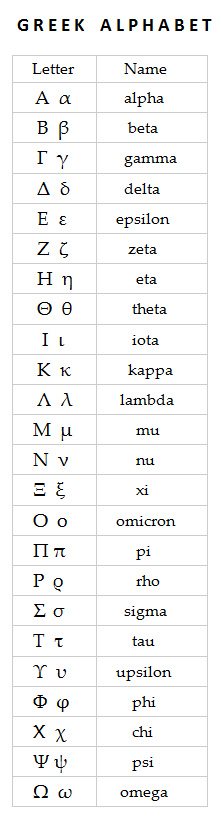# Mathematical symbols dictionary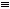–  identically equal,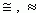–  approximately equal,

~  –  approximately,

0.(12345)  –  the repeating decimal with the period 12345,

N – the set of natural numbers,

Z –  the set of whole numbers (integers),

R –  the set of real numbers,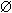–  an empty set,–  an infinity sign,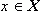–  an element x belongs to a set X ,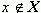–  an element x doesn't belong to a set X ,

X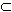Y –  a set X is a subset of a set Y ,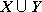–  a union of sets X and Y ,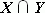–  an intersection of sets X and Y ,

X \ Y –  a symmetric difference of sets X and Y ,

{ u n } –  a sequence with a general term u n ,

[ a , b ]  –  a numerical segment,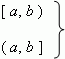–  numerical semi-segments (semi-intervals),

( a , b )  –  a numerical interval,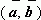–  a scalar product of vectors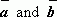,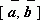–  a vector product of vectors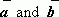,

==>   –  it follows,

<=>   –  equivalent,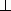–  perpendicular,–  parallel,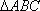–  a triangle ABC,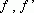–  a function and its derivative.